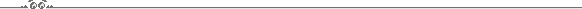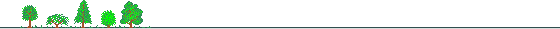Wise Home | Utilities

## RefractionWhile the light from celestial object is passes throgh the Earth atmosphere it is refracted by the air, the refraction depends on the light path distance, temperature, atmospheric pressure, etc. The net change of the refraction is to lower the apparent altitude of the celestial objects (relative to its geometric position).
The refraction of an object observed at the zenith is 0, while the refraction of an object observed near the horizon is about 34 arcmin.
Refraction correction formulae
approximate formulae for refraction correction for objects with zenith distance of up to 75o (altitude > 15o) :
R = 0o.00452 P / ((273 + T) tan a)
and for object below altitude of 15o:
R = P (0.1594 + 0.0196a + 0.00002a2)/[(273+T)(1 + 0.505a + 0.0845a2)]
Where P is the barometric pressure in millibars, T is the temperature in oC, a is the altitude measured in degrees and R is thr amount of refraction measured in degrees.

## Refraction calculator

 Altitude o Temperature oC Pressure millibars

Refraction arcmin.Constructed: November 1998, by: Eran Ofek